VK3CPU - Magloop Antenna Calculator Equations:

For single-turn circular loop inductance: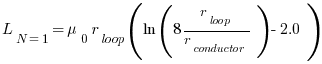[r_loop - loop radius in meters; r_conductor - conductor radius in meters]

For multi-turn circular loop inductance: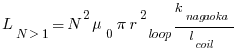[k_nagaoka - Nagaoka coefficient; l_coil - length of the coil in meters; r_loop - radius of the loop in meters]

For octagonal loop antenna inductance:[F W Grover]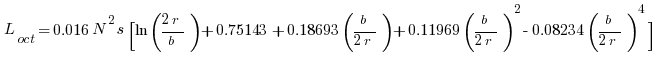[L - microhenries; N - turns; s - section side length cm; r - loop circumradius in cm; b - coil length in cm]

For hexagonal loop antenna inductance:[F W Grover]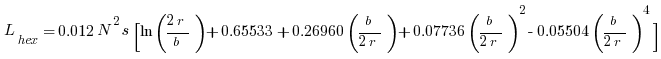[L - microhenries; N - turns; s - section side length cm; r - loop circumradius in cm; b - coil length in cm]

For square loop antenna inductance:[F W Grover]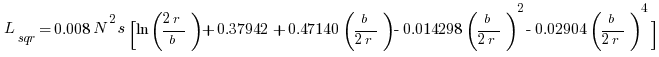[L - microhenries; N - turns; s - section side length cm; r - loop circumradius in cm; b - coil length in cm]

Loss resistance:Surface resistance: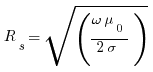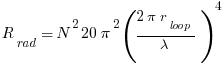Radiation resistance for octagon, hexagon and square loop: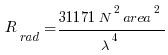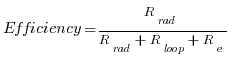Q factor: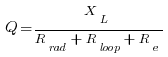Capacitor voltage: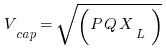Loop current: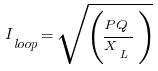Bandwidth: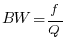Single-loop capacitance: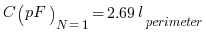[l_perimeter - conductor perimeter length in meters]

Multi-turn loop capacitance: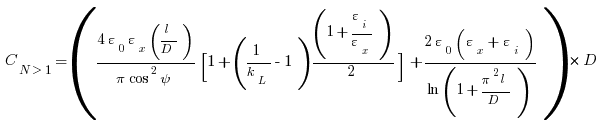[Based on David W Knight's (G3YNH) paper "The self-resonance and self-capacitance of solenoid coils: applicable theory, models and calculation methods"]